Author: Oscar Cronquist Article last updated on May 02, 2022## 1. How to use the LET function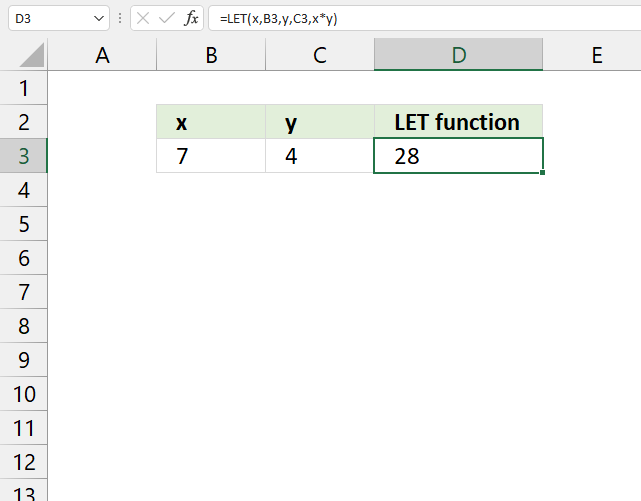The LET function lets you name intermediate calculation results which can shorten formulas considerably and improve performance. It can store up to 126 calculations and supports up to 126 names.

Formula in cell D3:

=LET(x, B3, y, C3, x*y)

The LET function in cell D3 gives cell reference B3 the name x and C3 the name y. The last argument is the actual formula, in this case, the asterisk multiplies x to y. 7*4 equals 28.

This example shows you how to name a cell reference, however, you can also name calculations. There is really no benefit in this example to use the LET function, it doesn't shorten the formula or speeds up the calculations. It only demonstrates how it works in a simple way.

## 2. Excel Function Syntax

LET(name1, name_value1, calculation_or_name2, [name_value2, calculation_or_name3...])

## 3. Arguments

 name1 Required. A name to assign that starts with a letter. name_value1 Required. The value that is assigned to name1, can be a constant, array, cell reference, or calculation (formula). calculation_or_name2 Required. It can be one of these things: calculation - A calculation that uses the specified names given in the LET function. name2 - Another name to assign. name_value2 Optional. The value that is assigned to name2, can be a constant, array, cell reference, or calculation (formula). calculation_or_name3 Optional. It can be one of these things: calculation - A calculation that uses the specified names given in the LET function. name3 - Another name to assign, name_value3 must be specified and so on.

The greatest benefit of using the LET function is if you use the same expression over and over in a formula. The LET function lets you calculate the expression once and then use the result in the formula as many times as you like.

This speeds up calculations and makes the formula shorter, sometimes much shorter. See the links (section 6) below for examples.

Tip! Give intermediate calculations descriptive names, this means that you don't need to remember what cell references point to or what calculations calculate, the name tells you what you need to know.

## 6. Examples

### 6.1 Example 1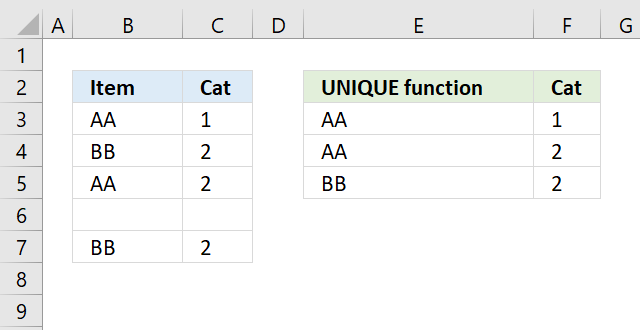This example demonstrates a formula that extracts unique distinct rows (records) sorted from A to Z ignoring blank rows. The LET function names an intermediate calculation x, the calculation is used three times in the formula.

This makes the formula much smaller, much faster to calculate, and easier to read and understand.

Formula in cell E3:

=LET(x, UNIQUE(FILTER(B3:C7, (C3:C7<>"")*(B3:B7<>"")), FALSE), SORTBY(x, INDEX(x, 0, 1), , INDEX(x, 0, 2), ))

Original formula:

=SORTBY(UNIQUE(FILTER(B3:C7, (C3:C7<>"")*(B3:B7<>"")), FALSE), INDEX(UNIQUE(FILTER(B3:C7, (C3:C7<>"")*(B3:B7<>"")), FALSE), 0, 1), , INDEX(UNIQUE(FILTER(B3:C7, (C3:C7<>"")*(B3:B7<>"")), FALSE), 0, 2), )

The following expression is repeated three times in the formula, I am naming it x:

x - UNIQUE(FILTER(B3:C7, (C3:C7<>"")*(B3:B7<>"")), FALSE)

### 6.2 Example 2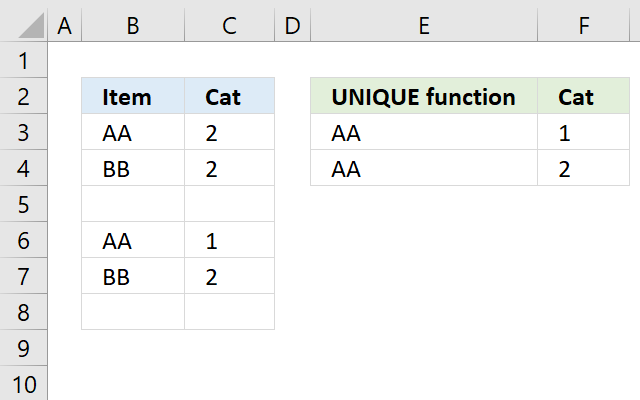This example demonstrates how to extract unique rows sorted from A to Z ignoring blank rows. The LET function names an intermediate calculation that is used three times in the calculation.

This makes the formula much smaller and much faster to calculate.

Formula in cell E3:

=LET(x, UNIQUE(FILTER(B3:C8, (C3:C8<>"")*(B3:B8<>"")), , TRUE), SORTBY(x, INDEX(x, 0, 1), , INDEX(x, 0, 2), ))

Original formula:

=SORTBY(UNIQUE(FILTER(B3:C8, (C3:C8<>"")*(B3:B8<>"")), , TRUE), INDEX(UNIQUE(FILTER(B3:C8, (C3:C8<>"")*(B3:B8<>"")), , TRUE), 0, 1), , INDEX(UNIQUE(FILTER(B3:C8, (C3:C8<>"")*(B3:B8<>"")), , TRUE), 0, 2), )

UNIQUE(FILTER(B3:C8, (C3:C8<>"")*(B3:B8<>"")), , TRUE) is repeated three times in the original formula. I am naming the intermediate expression x:

x - UNIQUE(FILTER(B3:C8, (C3:C8<>"")*(B3:B8<>"")), , TRUE)

The formula is now much shorter.

LET(x, UNIQUE(FILTER(B3:C8, (C3:C8<>"")*(B3:B8<>"")), , TRUE), SORTBY(x, INDEX(x, 0, 1), , INDEX(x, 0, 2), ))

Link to article: Extract unique rows sorted from A to Z ignoring blank rows

### 6.3 Example 3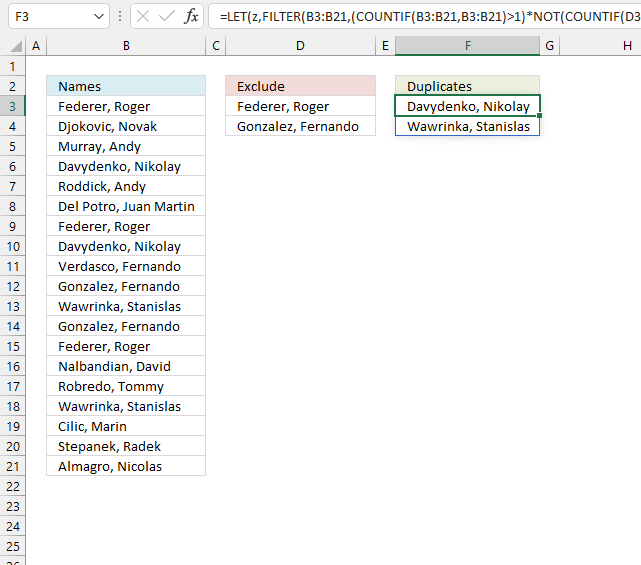This example shows a formula in cell F3 that extracts duplicate values from a column and excludes given values specified in column D.

The LET function names an intermediate calculation z which is repeated four times in the formula.

Formula in cell F3:

=LET(z, FILTER(B3:B21, (COUNTIF(B3:B21, B3:B21)>1)*NOT(COUNTIF(D3:D4, B3:B21))), FILTER(z, MATCH(z, z, 0)<>SEQUENCE(ROWS(z))))

This formula can be shortened even further:

=LET(x, B3:B21,z, FILTER(x, (COUNTIF(x, x)>1)*NOT(COUNTIF(D3:D4, x))), FILTER(z, MATCH(z, z, 0)<>SEQUENCE(ROWS(z))))

Original formula:

=FILTER(FILTER(B3:B21, (COUNTIF(B3:B21, B3:B21)>1)*NOT(COUNTIF(D3:D4, B3:B21))), MATCH(FILTER(B3:B21, (COUNTIF(B3:B21, B3:B21)>1)*NOT(COUNTIF(D3:D4, B3:B21))), FILTER(B3:B21, (COUNTIF(B3:B21, B3:B21)>1)*NOT(COUNTIF(D3:D4, B3:B21))), 0)<>SEQUENCE(ROWS(FILTER(B3:B21, (COUNTIF(B3:B21, B3:B21)>1)*NOT(COUNTIF(D3:D4, B3:B21))))))

The following intermediate calculation is repeated four times, I am naming it z:

z - FILTER(B3:B21, (COUNTIF(B3:B21, B3:B21)>1)*NOT(COUNTIF(D3:D4, B3:B21)))

Cell reference B3:B21 is repeated four times, I am naming it x:

x - B3:B21

Link to article: Extract duplicate values without exceptions - Excel 365

### 6.4 Example 4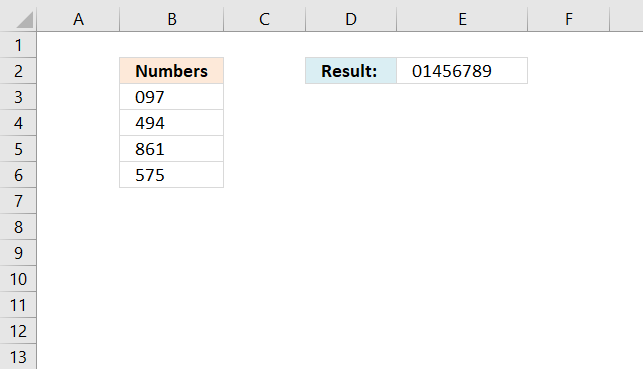This example demonstrates how to sort single digits from a cell range. An intermediate calculation is named x and is repeated two times in the calculation.

Formula in cell E2:

=LET(x, CONCAT(B3:B6), TEXTJOIN(, TRUE, SORT(UNIQUE(MID(x, SEQUENCE(LEN(x)), 1)))))

Original formula:

=TEXTJOIN(, TRUE, SORT(UNIQUE(MID(CONCAT(B3:B6), SEQUENCE(LEN(CONCAT(B3:B6))), 1))))